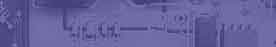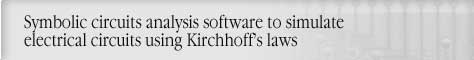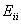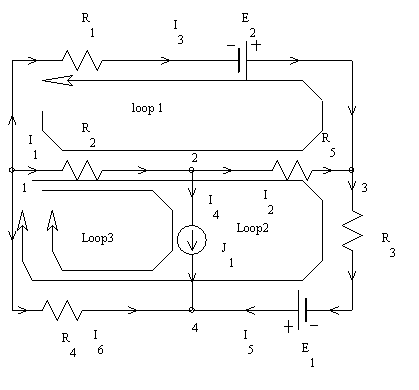Mesh Current circuit analysis method. Mesh Current circuit analysis using Circuit-MagicCircuit analysis Tutorial
AKNM Circuit Magic- circuit analysis software

Mesh Current Method

The mesh current method is deduced from the Kirchhoff’s voltage law (KVL) and superposition theorem.

The following formulas are used to solve circuit.whereLoop i resistance is the sum of resistances of all branches which contain in the given loop.Loop i to Loop j resistance. If the directions of loops are opposite then resistance is negative. Otherwise, it's positive. The value equals the sum of branches resistances of all branches, which contains in both loops.Loop i – EMF

Number of loop equations equal number of KVL equations.

Loop Number = Branch Number –(Nodes Number –1) – Current sources Number

If the circuit contain current sources it is necessary to create additional loops. Branch with current source can be contained in one loop.

Sample: Circuit Solution By Mesh Current

Step 1. Construct circuit using AKNM Circuit MagicElectrical scheme

Initial variables

R1=10Ohm; R2=20Ohm; R3=25Ohm; R4=10Ohm; R5=10Ohm;

E1=10V; E2=15V;

J1=10A;

Solution

I11·R11+I22·R12+I33·R13=E11

I11·R21+I22·R22+I33·R23=E22

(Loop 1 Resistance)

R11=R2+R5+R1=40

(Loop 1 to loop 2 Resistance is the sum of branch 1 and branch2 resistances. The resistance is positive due non-opposite Loop1 – Loop3 directions)

R12=R2+R5=30

R13=R2=20

R21=R2+R5=30

R22=R2+R5+R3+R4=65

R23=R2+R4=30

E11= -E2-I33·R31=-215

E22= E1-I33·R32=-290

40I11+30I22=-215

30I11+65I22=-290

I11=-3,102941

I22=-3,029412

I33=10

I1= I11+I22+I33=3,8676471

I2= I11+I22=-6,1323529

I3=-I11=3,1029412

I4= J1=10

I5= I22=-3,0294118

I6=-I22-I33=-6,9705882